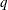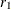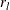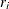### REQUIRES Statement

`REQUIRES` resource_constraint-1 <…resource_constraint-n> ;

where resource_constraint is specified in the following form:

activity_specification = (resource_specification) <QTY =>

where

activity_specification: (activity | activity-1 <…activity-m>)

and

resource_specification: (resource-1 <QTY => <…(,OR) resource-<QTY =>>)

activity_specification is a single activity or a list of activities that requiresunits of the resource identified in resource_specification. resource_specification is a single resource or a list of resources, representing a choice of resource, along with the equivalent required quantities for each resource. The default value ofis 1. Alternate resource requirements are separated by a comma (,) or the keyword OR. The QTY= parameter outside the resource_specification acts as a multiplier to the QTY= parameters inside the resource_specification.

The REQUIRES statement defines the potential activity assignments with respect to the pool of resources. If an activity is not defined, the REQUIRES statement implicitly defines the activity.

You can also define resource constraints by using the `Activity` and `Resource` data sets in lieu of, or in conjunction with, the REQUIRES statement. Any resource constraints that are defined for an activity by using a REQUIRES statement override all resource constraints for that activity that are defined by using the Activity and Resource data sets.

The following statements illustrate how you would use a REQUIRES statement to specify that activity A requires resource R:

``` activity A;
resource R;
requires A = (R);
```

In order to specify that activity A requires two units of the resource R, you would add the QTY= keyword as in the following example:

```requires A = (R qty=2);
```

In certain situations, the assignment might not be established in advance and there might be a set of possible alternates that can satisfy the requirements of an activity. This scenario can be defined by using multiple resource-specifications separated by commas or the keyword OR. For example, if the activity A needs either two units of the resource R1 or one unit of the resource R2, you could use the following statement:

``` requires A = (R1 qty=2, R2);
```

The equivalent statement using the keyword OR is

``` requires A = (R1 qty=2 or R2);
```

It is important to note that resources specified in a single resource constraint are disjunctive and not conjunctive. The activity is satisfied by exactly one of the resources rather than a combination of resources. For example, the following statement specifies that the possible resource assignment for activity A is either four units of R1 or two units of R2:

``` requires A = (R1 qty=2 or R2) qty=2;
```

The preceding statement does not, for example, result in an assignment of two units of the resource R1 and one unit of R2.

In order to model conjunctive resources by using a REQUIRES statement, such as when an activity requires more than one resource simultaneously, you need to define multiple resource constraints. For example, if activity A requires both resource R1 and resource R2, you can model it as follows:

```requires A = (R1)  A = (R2);
```

or

```requires A = (R1);
requires A = (R2);
```

If multiple activities have the same resource requirements, you can use an activity list for specifying the constraints instead of having separate constraints for each activity. For example, if activities A and B require resource R1 or resource R2, the specification

```requires (A B) = (R1, R2);
```

is equivalent to

```requires A = (R1, R2);
requires B = (R1, R2);
```